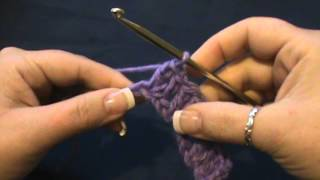#### How to Crochet the "Twin V Stitch"

##### Transcript:

[0.299]
yes welcome back we are going to learn how to do what's called a twin V stitch today and of course this is not difficult but you don't always have some of the simpler stitches stuck in your mind for some reason so I like to show you guys many many different stitches so what I did is I chained 18 and then we're going to start with this you're going to do two double crochets and into the v chain okay so count one two three four five all right I want you to put in two double crochets here

[40.469]
okay and then what I want you to do is to double crochet into the next chain

[51.649]
you're going to skip two chains okay and you're going to put two double crochet into each of the next two so we're going to have a total of four two in this stitch and two in the next

[75.4]
okay and then you're going to repeat that so we're going to skip two chains on to and then you're going to do two double crochet into each of the next two so you'll do that across your row so there's two in that one two in the next one okay you'll skip the next two and that should leave you with two let's get that in there let's do two in the next

[126.25]
and to in the next

[133.45]
all right this point this is what you should have you've got three space gaps in between there now let's chain three and we're going to turn now you're going to skip two stitches okay and then you're going to do two double crochet in each of the next two stitches so you're going to skip two stitches so one two one two and then you're going to put two stick two double crochet into this this next stitch right here and then into this next one so you're just making double stitches all the way through this pattern okay

[187.849]
so this is what you should have so far and then you're just going to repeat that so you're going to skip to and then you know skip those two basically it's the second group of two double crochet that you did on the first row remember we did two and then a space and then two you're doing it in the second group I'm sorry guys hmm that's the second time done that okay skip to

[231.13]
do two double crochet in the next two double crochet okay so this is what it should be looking like okay and then you're going to skip the next two and then two double crochet in the next two

[259.28]
and then you're gonna finish off with a double crochet here at the end okay so see there's nothing difficult about it and if you see right here where I was telling you down here at the bottom where we did this set of two double crochets in this set and there's a space there just just a little space you're working over the top of the second set and then you're going to come here and you're going to chain three and you're going to do the same thing you're going to go back and repeat row two but you're going to skip the first two here and then come here and do them okay so it that's just a little bit different of a pattern and I thought it was very simple for some of our new crochets out there so I wanted to give you an option as for a pattern in case you were wondering so again this was the twin V stitch okay all right guys have a great day until